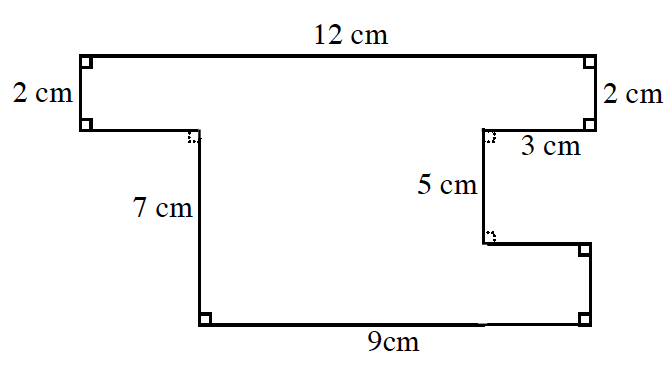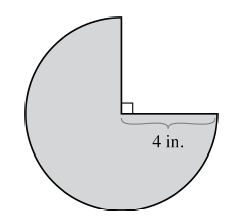### Home > CC3 > Chapter 4 > Lesson 4.1.6 > Problem4-62

4-62.

Find the area and perimeter of each shape. Show your work.

1.Divide this shape into smaller rectangles and find the areas of these.

$\text{A }=72$ sq cm

To find the perimeter, add the lengths of all the sides.

$2+12+2+3+5+3+2+9+7+3=48\text{ cm}$

1.Find the area of a circle $\pi r^2$. Notice that this is only three-fourths of a circle.

Find the circumference of a circle ($2\pi r$). Note that this is, again, three-fourths of a circle.
Once you find the adjusted circumference, remember to incorporate the radius of the straight edges in your calculations.